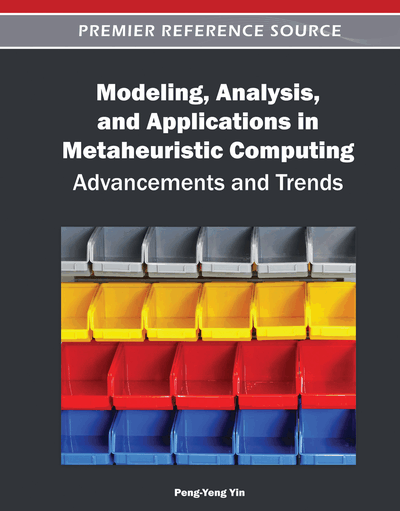# Stochastic Learning for SAT- Encoded Graph Coloring Problems

Noureddine Bouhmala (Vestfold University College, Norway) and Ole-Christoffer Granmo (University of Agder, Norway)
Copyright: © 2012 |Pages: 20
DOI: 10.4018/978-1-4666-0270-0.ch017
OnDemand PDF Download:
Available
\$37.50
No Current Special Offers

## Abstract

The graph coloring problem (GCP) is a widely studied combinatorial optimization problem due to its numerous applications in many areas, including time tabling, frequency assignment, and register allocation. The need for more efficient algorithms has led to the development of several GC solvers. In this paper, the authors introduce a team of Finite Learning Automata, combined with the random walk algorithm, using Boolean satisfiability encoding for the GCP. The authors present an experimental analysis of the new algorithm’s performance compared to the random walk technique, using a benchmark set containing SAT-encoding graph coloring test sets.
Chapter Preview
Top

## 1. Introduction

In the graph coloring problem (GCP) an undirected graph G(V, E) is given, where V is a set of vertices, and E is a set of pairs of vertices called edges. We call a k-coloring of G, a mappingsuch that if C(p) = C(q) then. The setis the set of colors. There exist two variants of this problem. In the optimization variant, the goal is to find the chromatic number X(G), which is the minimal k for which there exists a k-coloring of G. In the decision variant, the question is to decide whether for a particular number of colors, a coloring of G exists. All these problems are known to be NP-complete, so it is unlikely that a polynomial-time algorithm exists that solves any of these problems.

In this paper the focus is on the decision variant of the GCP. Encoding the GCP as a Boolean satisfiability problem (SAT) and solving it using efficient SAT algorithms has caused considerable interest. The SAT problem, which is known to be NP-complete (Cook, 1971), can be defined as follows. A propositional formulawith m clauses and n Boolean variables is given. A Boolean variable is a variable that can take one of the two values, True or False. Each clause, in turn, has the form:

whereanddenotes the negation of. The task is to determine whether the propositional formula Φ evaluates to True. Such an assignment, if it exists, is called a satisfying assignment for Φ, and Φ is called satisfiable. Otherwise, Φ is said to be unsatisfiable. Most SAT solvers use a Conjunctive Normal Form (CNF) representation of the propositional formula. In CNF, the formula is represented as a conjunction of clauses, where each clause is a disjunction of literals, and a literal is a Boolean variable or its negation. For example, PQ is a clause containing the two literals P and Q. This clause is satisfied if either P is True or Q is True. When each clause in Φ contains exactly k literals, the resulting SAT problem is called k-SAT.

The paper is organized as follows. In Section 2, we review various algorithms for solving GCP, as well as satisfiability algorithms for solving SAT-encoded GCP. Section 3 explains the basic concepts of Learning Automata (LA) and introduces our new LA based approach to graph coloring. In Section 4, we look at the results from testing the new approach and draw some conclusions. Finally, in Section 5 we present a summary of the work.

## Complete Chapter List

Search this Book:
Reset